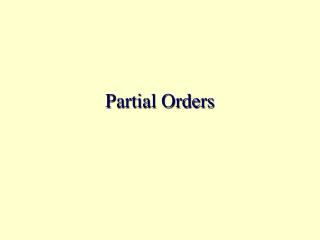DownloadDownload PresentationPartial Orders

# Partial Orders

Download Presentation## Partial Orders

- - - - - - - - - - - - - - - - - - - - - - - - - - - E N D - - - - - - - - - - - - - - - - - - - - - - - - - - -
##### Presentation Transcript

1. Partial Orders

2. Definitions • A relation R on set A is a partial order if it is: • reflexive • antisymmetric • transitive. • A is called a partially ordered set or poset. • [A;R] means A is partially ordered by R. • Example: [ P({1,2});  ] (draw its digraph).

3. Definitions ... • is the prototype of a partial order • a, bA are comparable under  if either ab or ba. • Otherwise, they are said to be incomparable • If a, bA are comparable, then [A; ] is totally ordered and A is a chain.

4. Examples • [ Z;  ], • where Z is the set of integers •  has the usual meaning. • Z is totally ordered. • [ Z+; | ], • where Z+ is a set of positive integers • | is the (evenly) divides operator (e.g., 2 | 10 )

5. Hasse Diagrams • A Hasse diagram is a directed graph (digraph), where • self-loops are omitted • arcs implied by transitivity are omitted. • Let Dn denote the set of positive divisors of n. • Draw Hasse diagram for [ D8; | ] • Draw Hasse diagram for [ D6; | ]

6. Hasse Diagrams • What n yields a poset [Dn; | ] whose Hasse diagram is a cube of dimension: • 0 • 1 • 2 • 3

7. Examples • Let S be a set. [ P(S);  ] is a poset. • Draw the Hasse diagram for: • S =  • S = {1} • S = {1, 2} • S = {1, 2, 3} • What do you think the Hasse diagram looks like for S = {1, 2, 3, 4}? For S = {1, . . ., n}?

8. Computer Science Example • Consider this sequence of Java assignments: a = b + d + c; d = a*(b + d) + c; e = (b + d)*c; (Draw an operator graph for these statements.) • Sequence these operations in a way that is compatible with their partial order. • Where are the longest paths?

9. Topological Sorting • Let G = (V,E) be a directed graph where • v V represents a task; • (u, v)  E means that task u must be completed before v can be started. • G cannot have cycles. • Problem: Find a schedule for G that respects the partial order.

10. Composing Relations • Let R be a relation from A to B. • Let S be a relation from B to C. • The composition of R & S, denoted RS, is RS = { xRSy | zB, xRz  zSy }. • Example: • R = { (1,2), (3,4), (2,4) } • S = { (2,4), (2,3), (4,1) } • RS = { (1,4), (1,3), (3,1), (2,1) }

11. The Transitive Closure • Let R be a relation from S to S. • RR is usually denoted R2. • Ri+1 = RiR. • The transitive closure, R+, of a binary relation R is:

12. Reflexive-Transitive Closure • R0 = { (x, x) | x  S } • The reflexive-transitive closure, denoted R*, is: R* = R0  R+. That is,

13. Example R a b c d e R0 Self-loops not shown a b c d e R+ a b c d e R* Self-loops not shown a b c d e